Published: 07 September 2023

# Automatic vibration control method for grasping end of flexible joint robot

Yufang Sun1
1College of Mechanical and Electrical Engineering, Heilongjiang Institute of Technology, Harbin, 150050, China
Article in Press
Views 39

#### Abstract

Because flexible robots have flexible components such as reducers, there are problems of accuracy deviation and end vibration in the process of external interference and trajectory tracking. This leads to the proposal of a Sliding Mode Control Approach Based on RBF Neural Network (SMC-RBF) parameter optimization. This method is mainly applied to reduce the end vibration and running position error of flexible robot. Firstly, the Newton-Euler method is used to establish the dynamic model of robot considering joint flexibility. At the same time, the experiment optimizes the Sliding Mode Control (SMC) method through RBF neural network. The experiments verify the control methods of the two-joint flexible robot and the six-joint flexible robot respectively. In the control of two-joint robot, the maximum tracking curve error of SMC is only about 0.25 rad under the interference of pulse signal; And the recovery time is only about 1 s. In the control of 6-joint robot, the maximum error of RBF-sliding mode control method on $XYZ$ axis is 0.7 mm, 0.25 mm and 1.25 mm respectively; The error on three axes is smaller than that of traditional PD control method. The results demonstrate that the tracking error of the improved mode control is small, the chattering phenomenon of the robot system is weakened as well.

## 1. Introduction

With the rapid advancement of domestic robot technique, flexible cooperative robots have been widely applied in 3C electronics, medical, automotive parts integration and other industries due to their high load ratio, compact structure and low power consumption. However, flexible robots have strong rigid-flexible coupling characteristics. When subjected to external interference or excessive acceleration, tracking error and end vibration will occur . Vibration affects the efficiency of robots in industrial production. Therefore, suppressing the vibration of flexible manipulator and improving the positioning accuracy become an important subject . Many models have been suggested for the flexible robots at home and abroad, among which PID control, singular perturbation control and SMC are popular . In this study, the Newton-Euler method is applied to establish the robot dynamics model considering joint flexibility, so as to analyze the mathematical characteristics of the flexible robot dynamics model. Secondly, the experiment shows that the vibration and SMC of flexible robot require high model accuracy. Therefore, a method based on SMC-RBF error compensation is designed in this study. In this method, RBF algorithm is applied to compensate the modeling error in the control rate online, and the adaptive rate of neural network weight update is mainly derived by Lyapunov method. Finally, the performance of the optimal control method is analyzed by taking two-joint flexible robot and six-joint flexible robot as experimental objects. The research’s aim is to optimize the SMC to weaken the vibration of Flexible Joint Robots (FJB). At the same time, it also aims to enhance the control performance, improve the control accuracy, and improve the working efficiency of the robot in industrial production applications. The experiment aims to provide scientific reference for the progress of industrial robot automation technology.

## 2. Related work

In the control of multi-joint flexible robots, Datouo R. et al. proposed an adaptive fuzzy finite-time command filtering backstepping control method. The experiment shows that this method not only has low calculation cost, but also has good control effect and stable robot performance . Abdul-Adheem et al. proposed an input-output active feedback linearization technology to control a single-link flexible robot. This technology is mainly aimed at the anti-interference technology in the process of robot control. It is concluded that the model has closed-loop stability and can effectively eliminate control interference . Pham Minh-Nha et al. also studied the vibration control of six-joint flexible robot. In this paper, the author proposes a discrete controller and a method of optimizing control loop gain. In the experiment, this method performs well; It performs well in control anti-interference and vibration suppression . Singular perturbation method is one of the basic theories of this paper. Hooshmand H et al. also adopted singular perturbation method. The basic control method of model reduction optimization is adopted in the experiment to reduce the control complexity. It is obviously that the method has simple structure and stability . Zhang Q. and others seek vibration control methods for elastic deformation vibration of flexible robots. Therefore, an adaptive SMC algorithm is suggested. The results show that the three-axis error of the robot is reduced by 12.1 %, 38.8 % and 50.34 % . Yang H. J. et al. selected the radial basis function network to control the flexible robot, and used partial differential equations for model analysis in the experiment. The advantage of this method is that the controller only needs to measure boundary information, which is more convenient in engineering applications . Soltanpour et al. took the multi-DOF FJB as the research object, and constructed a control method for optimizing SMC through time-varying parameters. The experiment shows that the method ensures the global asymptotic stability of the closed-loop system .

In terms of SMC and RBF model, domestic and foreign scholars have rich application achievements. Li Fang et al. used SMC to pneumatic muscle actuator of flexible robot. The experiment achieves the goal of error control and anti-interference ability improvement based on the application of saturation function. Experiments show that the method is robust. The proposed method shows high stability in the trajectory tracking experiment . On the basis of dynamics theory, Fallah Zeinab et al. adopted Markov model to optimize the SMC method. In this paper, the parameters of sliding surface are optimized by RBF neural network and nominal model; The linear matrix inequality of Markov chain is used for optimization. The experiment shows that this method is also effective . Qin Qiuyue et al. also applied SMC method, but the experimental object was a bilaterally symmetric hybrid robot. The optimization by the proposed model is completed by compound error. At the same time, they confirmed the asymptotic convergence and stability of the error of the proposed method through experiments . Zijie Niu et al. combined RBF network with PID algorithm to propose a new type of Mecanum vehicle control method. The purpose of its research is to help the vehicle driver to correct the direction. The experiment shows that the improved control model reduces the correction time by 1.4 s . In the combination of machine control and RBF neural network, Jinxiang Wang et al. also proposed a driver-vehicle-road (DVR) model to describe the driver’s steering behavior. The purpose of this study is to realize human-machine shared steering control during driving .

To sum up, the research on the control of flexible robots at home and abroad has achieved certain results. Scholars generally recognize that the traditional SMC has the disadvantage of discontinuous control rate. Therefore, optimization is carried out in the direction of fuzzy algorithm, Markov model and composite error. However, the optimization results of RBF neural network are less, and its application in the field of production robot is also less. At the same time, in the current research results, improving work accuracy and stability often adopts the method of reducing motion speed, which greatly restricts the work efficiency of hybrid structure flexible robotic arms. In this paper, RBF and Sliding mode control are combined, and the unknown model of the system is approximated by the characteristics of universal approximation of neural network, so as to realize a control method that does not need an accurate model, which is expected to provide a reference for neural network optimal control.

## 3. Research on neural SMC of FJB grasping end vibration

### 3.1. Dynamic model construction of end vibration of FJB

The accurate dynamic model construction of FJB is the basis of achieving high-precision control and vibration suppression. For the analysis of dynamic model of FJB, Newton-Euler method is used for vector mechanics modeling in this study. Newton-Euler modeling is based on Newton’s equation and Euler’s equation. By analyzing the speed and acceleration of each link of the manipulator, the interaction force between each link is determined. At the same time, the experiment completed the derivation of the overall dynamic model of the robot through chain iteration. The Newton-Euler model clarifies the interaction forces between the links, which is more conducive to the design of control algorithms for flexible robots. Due to the complex content and structure of the flexible joint, the robot joint is equivalent to the linear torsion spring proposed by Spong. The equivalent model is shown in Fig. 1.

Fig. 1Equivalent model of robot flexible joint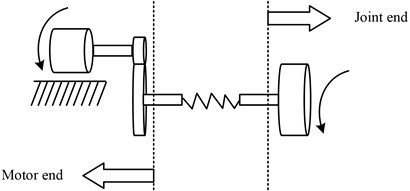It can be seen from Fig. 1 that the elastic force generated by joint flexibility and joint torsion form become linear. The proportional coefficient is the elastic coefficient of the spring. This model concentrates the mass of the rotor on the shaft. Before building the dynamic model of FJB, to simplify the mechanical structure and calculation process, the subsequent presumptions are first proposed: (1) The flexible joint is equivalent to the Spring model, that is, the linear torsion spring, and the spring force is linear with the joint shape variables. (2) Ignoring the influence of the motor rotor, it is assumed that the motor rotor is coaxial with the joint axis. (3) Without considering the coupling effect of motor dynamics and robot dynamics, it is assumed that the motor is a perfect source of torque and that its reaction time is adequate. (4) It is assumed that the mass of the connecting rod is uniformly distributed and the connecting rod will not deform, and the position of the connecting rod centroid is 1/2 of the length of the connecting rod. Set the ideal torque of motor output as ${\tau }_{motor,i}$; The torque changes to ${r}_{i}$ after the transmission of the reducer with a reduction ratio of ${\tau }_{l,i}$. The motor output angle is ${\theta }_{motor,i}$; The actual output angle is ${\theta }_{l,i}$ after decelerating by the reducer. The moment of inertia of the motor rotor is ${J}_{motor,i}$, and the moment of inertia after deceleration is ${J}_{l,i}$. The relationship between the three decelerator before and after conversion is expressed as the following Eq. (1):

1
$\left\{\begin{array}{l}{\tau }_{l,i}={r}_{i}\cdot {\tau }_{motor,i},\\ {\theta }_{l,i}=\frac{{\theta }_{motor,i}}{{r}_{i}},\\ {J}_{l,i}=\frac{{r}_{i}^{2}}{{J}_{motor,i}},\end{array}\right\$

where, $l$, $i$ all indicate the connecting rod number. When the joint stiffness is insufficient, the robot joint will undergo small deformation. That is, the connecting rod’s angle and the reducer’s output rotation angle are not equivalent., and its relationship is expressed as Eq. (2):

2
${\tau }_{i}={K}_{i}\left({\theta }_{m,i}-{q}_{i}\right),$

where, $i$ represents the number of flexible joints; ${\tau }_{i}$ indicates the driving torque of the $i$-th connecting rod; ${K}_{i}$ indicates the elastic coefficient of the torsion spring. ${q}_{i}$ indicates the actual angle of the $i$ connecting rod. As stated by the Newtonian dynamics formula $F=ma=m\frac{dv}{dt}$ and the description of rotation motion in Euler equation, the dynamic moment generated by rotation is expressed as Eq. (3):

3
$J=\sum _{i=1}^{n}\left({\rho }_{i}×{m}_{i}{v}_{i}\right)=I\omega ,$

where, ${\rho }_{i}$ denotes the radius of rotation; ${m}_{i}$ indicates the centroid mass; $\omega$ denotes the linear speed of the centroid rotation. ${v}_{i}$ is the center of mass's moment of inertia; $I$ represents the rotational angular speed of the centroid. Therefore, according to the theorem of moment of momentum, the resultant moment of the resultant force on the fulcrum is expressed as $M=\frac{dJ}{dt}=J\text{'}+\omega ×I\omega$. According to the recursive algorithm of Newton-Euler method, the extrapolation formula of velocity and acceleration on both banks is expressed as Eq. (4):

4
$\left\{\begin{array}{l}{}^{i+1}\mathrm{}{F}_{i+1}^{}={m}_{i+1}{}_{\mathrm{}}{}^{i+1}\mathrm{}{v\text{'}}_{Ci+1},\\ {}_{\mathrm{}}{}^{i+1}\mathrm{}{N}_{i+1}^{}={}_{\mathrm{}}{}^{Ci+1}\mathrm{}{I}_{i+1}{}_{\mathrm{}}{}^{Ci+1}\mathrm{}{\omega \text{'}}_{i+1}+{}_{\mathrm{}}{}^{i+1}\mathrm{}{\omega }_{i+1}×{}_{\mathrm{}}{}^{Ci+1}\mathrm{}{I}_{i+1}{\omega }_{i+1},\end{array}\right\$

where, ${}^{i+1}\mathrm{}{F}_{i+1}^{}$ represents the resultant force on the centroid of connecting rod $i+1$; ${}^{i+1}\mathrm{}{N}_{i+1}^{}$ is expressed as the resultant time on the centroid of the connecting rod $i+1$. ${}^{i+1}\mathrm{}{v\text{'}}_{Ci+1}$ expressed as the acceleration of the centroid of the connecting rod $i+1$; ${}^{Ci+1}\mathrm{}{I}_{i+1}$ represents the inertia tensor of the center of mass of the connecting rod $i+1$; ${\omega }_{i+1}$ and ${\omega \text{'}}_{i+1}$ respectively represent the angular velocity and angular acceleration of the connecting rod $i+1$. The connecting rod's driving power and torque are expressed in Eq. (5):

5
$\left\{\begin{array}{l}{}^{i}\mathrm{}{f}_{i}={}_{i+1}{}^{i}\mathrm{}{R}^{i+1}{f}_{i+1}+{}_{\mathrm{}}{}^{i}\mathrm{}{F}_{i},\\ {}_{\mathrm{}}{}^{i}\mathrm{}{n}_{i}={}_{\mathrm{}}{}^{i}\mathrm{}{N}_{i}+{}_{i+1}{}^{i}\mathrm{}{R}^{i+1}{n}_{i+1}+{}_{\mathrm{}}{}^{i}\mathrm{}{P}_{Ci}×{}_{\mathrm{}}{}^{i}\mathrm{}{F}_{i}+{}_{\mathrm{}}{}^{i}\mathrm{}{P}_{i+1}×{}_{i+1}{}^{i}\mathrm{}{R}^{i+1}{f}_{i+1}\\ {\tau }_{i}={}_{\mathrm{}}{}^{i}\mathrm{}{n}_{i}^{T}{}_{\mathrm{}}{}^{i}\mathrm{}{Z}_{i},\end{array}\right\,$

where, ${}^{i}\mathrm{}{f}_{i}$ and ${}^{i}\mathrm{}{n}_{i}$ represent the force and moment at joint $i$; ${\tau }_{i}$ is the driving torque of the joint. ${}_{i+1}{}^{i}\mathrm{}{R}^{i+1}$represented as the rotation matrix from coordinate system $i$ to coordinate system $i+1$; ${}^{i+1}\mathrm{}{Z}_{i+1}=\left[0,0,1{\right]}^{T}$, ${}^{i}\mathrm{}{P}_{i+1}$ represents the position of the coordinate system $i+1$ relative to the coordinate system $i$; ${}^{i}\mathrm{}{P}_{Ci}$ represented as the centroid position of the connecting rod $i$. Finally, improve the friction term and joint inertia in the dynamic model, and Eq. (6) shows it:

6
$\left\{\begin{array}{l}{\tau }_{F}={f}_{v}{\theta \text{'}}_{m}+{f}_{c}\mathrm{s}\mathrm{g}\mathrm{n}\left({{\theta }^{\text{'}}}_{m}\right),\\ {\tau }_{J}=J{\theta \text{'}\text{'}}_{m},\end{array}\right\$

where, ${\tau }_{F}$ represents the friction model; ${\tau }_{J}$ represents joint inertia force. ${f}_{v}$ represents viscous friction, and ${f}_{c}$ represents coulomb friction. Finally, the dynamic model of the FJB can be expressed by combining the Eq. (2):

7
$\left\{\begin{array}{l}M\left(q\right){q}^{\text{'}\text{'}}+C\left(q,{q}^{\text{'}}\right){q}^{\text{'}}+G\left(q\right)+{\tau }_{d}=\tau ,\\ K\left({\theta }_{m}-q\right)=\tau ,\\ J{\theta \text{'}\text{'}}_{m}+\tau ={\tau }_{m}-{\tau }_{F},\end{array}\right\$

where, $M\left(q\right)$ denotes the robot’s connecting rod’s inertia matrix; $C\left(q,q\text{'}\right)$ is the centrifugal force matrix; $G\left(q\right)$ indicates the gravity vector; ${\tau }_{d}$ denotes the uncertain interference term of robot joint; $\tau$ denotes the torque of the connecting rod. To apply the property expression, after building the dynamic model of the FJB, the research will express the dynamic characteristics of the robot through the mathematical model. In the feature of parameter linearization, set the function $n*m$ with $Y\left(q,q\text{'},q\text{'}\text{'}\right)$ and the dimension vector $m$ of $\mathrm{\Theta }$, so that the robot dynamics formula can be written into Eq. (8):

8
$M\left(q\right){q}^{\text{'}\text{'}}+C\left(q,{q}^{\text{'}}\right){q}^{\text{'}}+G\left(q\right)=Y\left(q,{q}^{\text{'}},{q}^{\text{'}\text{'}}\right)\mathrm{\Theta }.$

### 3.2. Optimization of SMC based on RBF neural network error compensation

The vibration control of conventional FJB adopts PID control, sliding mode variable structure control, singular perturbation method, neural network control, adaptive control, etc. In this research, to improve SMC for vibration suppression, a neural network is utilized. The chattering effect of SMC is often caused by the modeling error of the system and the sign function in the control rate. Therefore, to eliminate the chattering phenomenon, it is required to consider two aspects: compensating the modeling error and changing the control rate structure. In this study, RBF model is used to enhance SMC. RBF neural network universal approximation principle is used to compensate the modeling error of the system online.

Fig. 2RBF neural network optimization process for SMC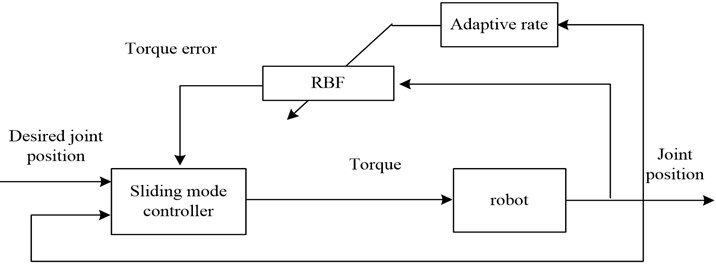As shown in Fig. 2, in this vibration suppression method, the optimization of SMC mainly uses Lyapunov method to derive the neural network weight adaptive rate. In the experiment, RBF neural network is used for data set training, and the goal of stable convergence of the control system is finally achieved. First, the position error is defined as $e\left(t\right)={q}_{d}\left(t\right)-q\left(t\right)$; The velocity error is $e\text{'}\left(t\right)={q\text{'}}_{d}\left(t\right)-q\text{'}\left(t\right)$. Therefore, the system modeling error is expressed as Eq. (9) by combining Eq. (8):

9
$\mathrm{\Delta }\tau =\left(M-\stackrel{^}{M}+J-\stackrel{^}{J}\right)\left({e}^{\text{'}\text{'}}+\beta {e}^{\text{'}}+{q}^{\text{'}\text{'}}\right)+\left(C-\stackrel{^}{C}\right)\left({e}^{\text{'}}+\beta e+{q}^{\text{'}}\right)+\left(G-\stackrel{^}{G}\right)+\left({\tau }_{F}-{\stackrel{^}{\tau }}_{F}\right),$

where, the system error is related to the actual angle $q$, actual angular velocity $q\text{'}$, actual angular acceleration $q\text{'}\text{'}$, joint angular error $e$, joint angular speed error $e\text{'}$, and joint angular acceleration error $e\text{'}\text{'}$ of the robot joint. Set the above parameters as the input of the neural network, namely $x=\left[{e}^{T},{e\text{'}}^{T},{e\text{'}\text{'}}^{T},{q}^{T},{q\text{'}}^{T},{q\text{'}\text{'}}^{T}\right]$. If the RBF network is applied to compensate the system modeling deviation, the network output under ideal conditions is expressed as Eq. (10):

10
$\left\{\begin{array}{l}{\varphi }_{i}\left(x\right)=\frac{h\left({‖x-{c}_{i}‖}^{2}\right)}{{b}_{i}^{2}},\\ \mathrm{\Delta }\tau =W\mathrm{\Phi }\left(x\right)+\mathrm{\Delta }E,\end{array}\right\$

where, $W$ denotes the network’s ideal weight matrix; $\mathrm{\Phi }\left(x\right)$ is radial basis function’s output; $\mathrm{\Delta }E$ indicates the error of neural network. Assume that RBF’s true output is $\mathrm{\Delta }\stackrel{^}{\tau }=W\mathrm{\Phi }\left(x\right)$. Take $\stackrel{-}{W}=W-\stackrel{^}{W}$ to represent the deviation between the true weight and the ideal weight; Then the SMC law of the robot can be set as Eq. (11) based on the nominal model ${\tau }_{s}=\mathrm{\Psi }+\lambda s+\sigma \mathrm{s}\mathrm{g}\mathrm{n}\left(s\right)$:

11
${\tau }_{s}=\mathrm{\Psi }+\lambda s+{\stackrel{^}{W}}^{T}\mathrm{\Phi }\left(x\right)+{k}_{\sigma }\mathrm{s}\mathrm{g}\mathrm{n}\left(s\right),$

where, $\mathrm{\Psi }$ represents the nominal model; (A $s\left(t\right)=e\text{'}+\beta e$) is the error description function; $\lambda$ is the weight of convergence rate; $\sigma$ is the weight of robot vibration. ${k}_{\sigma }$ represents the resilient term coefficient to cancel the system's uncertain disturbance. To derive the weight adaptive rate, the Lyapunov function is defined as: $V=\frac{1}{2}{s}^{T}\left(M+J\right)s+\frac{1}{2}tr\left({\stackrel{-}{W}}^{T}{\mathrm{\Gamma }}^{-1}\stackrel{-}{W}\right)$. There are:

12
${V}^{\text{'}}={s}^{T}\left(M+J\right){s}^{\text{'}}+\frac{1}{2}{s}^{T}{M}^{\text{'}}s+tr\left({\stackrel{-}{W}}^{T}{\mathrm{\Gamma }}^{-1}\stackrel{-}{{W}^{\text{'}}}\right)={s}^{T}\left(M+J\right){s}^{\text{'}}+{s}^{T}Cs+tr\left({\stackrel{-}{W}}^{T}{\mathrm{\Gamma }}^{-1}\stackrel{-}{{W}^{\text{'}}}\right),$

where, $tr\left(\right)$ represents the trace of the matrix; $\mathrm{\Gamma }$ represents a matrix of symmetric positive definite constants. As stated by the characteristics of the robot dynamics model built in the previous section, it can be concluded that: ${x}^{T}\left[M\text{'}\left(q\right)-2C\left(q,q\text{'}\right)\right]x=0$, so the Lyapunov function can be modified to Eq. (13):

13
${V}^{\text{'}}={s}^{T}\left[\mathrm{\Psi }+\Delta \tau -{\tau }_{s}+{\tau }_{d}\right]+tr\left({\stackrel{-}{W}}^{T}{\mathrm{\Gamma }}^{-1}\stackrel{-}{{W}^{\text{'}}}\right).$

Substituting neural SMC law Eq. (11) into Eq. (13), the following Eq. (14) can be obtained:

14
$\begin{array}{l}V\text{'}={s}^{T}\left[\stackrel{-}{W}\mathrm{\Phi }\left(x\right)+\Delta E-\lambda s-{k}_{\sigma }\mathrm{s}\mathrm{g}\mathrm{n}\left(s\right)+{\tau }_{d}\right]+tr\left({\stackrel{-}{W}}^{T}{\mathrm{\Gamma }}^{-1}\stackrel{-}{W\text{'}}\right)\\ =-{s}^{T}\lambda s+{s}^{T}\left[-{k}_{\sigma }\mathrm{s}\mathrm{g}\mathrm{n}\left(s\right)+\Delta E+{\tau }_{d}\right]+{s}^{T}\left[\stackrel{-}{W}\mathrm{\Phi }\left(x\right)\right]+tr\left({\stackrel{-}{W}}^{T}{\mathrm{\Gamma }}^{-1}\stackrel{-}{{W}^{\text{'}}}\right).\end{array}$

It can be seen from the circular nature of the matrix trace that when there is a symmetric relationship between different matrices of the same dimension, the trace of their scores will not change under all the rings; At the same time, when the product of all cyclic sequences still exists, their traces will still be identical, but only satisfy the product of cyclic positions, not the product of all $p$:

15
${V}^{\text{'}}=-{s}^{T}\lambda s+{s}^{T}\left[-{k}_{\sigma }\mathrm{s}\mathrm{g}\mathrm{n}\left(s\right)+\Delta E+{\tau }_{d}\right]+tr\left({\stackrel{-}{W}}^{T}\left({\mathrm{\Gamma }}^{-1}\stackrel{-}{{W}^{\text{'}}}+\mathrm{\Phi }\left(x\right){s}^{T}\right)\right).$

To make the derivative of Lyapunov function less than 0, take $\left\{\begin{array}{l}{k}_{\sigma }>‖\mathrm{\Delta }E‖+‖{\tau }_{d}‖\\ \stackrel{-}{W\text{'}}=-\mathrm{\Gamma }\mathrm{\Phi }\left(x\right){s}^{T}\end{array}\right\$. Once the neural network design is completed, $‖\mathrm{\Delta }E‖$ will approach 0. Therefore, the value of ${k}_{\sigma }$ will be far less than the sliding and grinding switching coefficient in the traditional SMC law, thus achieving the effect of weakening the robot vibration. At the same time, the expected weight $\stackrel{-}{W}=W-\stackrel{^}{W}$ in $W$ is regarded as a constant. Therefore, the adaptive rate is expressed as Eq. (16):

16
$\stackrel{^}{W\text{'}}=\mathrm{\Gamma }\mathrm{\Phi }\left(x\right){s}^{T}.$

## 4. Optimization verification analysis of SMC based on RBF neural network error compensation

### 4.1. Simulation experiment of two-joint flexible robot under SMC

To test the control performance of the SMC strategy, experiments and practical application analysis are carried out on the two-joint flexible robot and the six-joint flexible robot respectively. First, in the experiment of two-joint flexible robot, set the control proportion parameter as [3000 2900] T; The coefficient of differential link is [250 120] T. Set the position error weight parameter as [20 2.34] T; The weight parameter of convergence speed is  T; The robot vibration weight parameter is [10 120] T. The simulation model is built through simulink in this experiment. The model of the experimental object of the two-joint robot is shown in Fig. 3.

Fig. 3Dynamic model of 2-joint flexible robot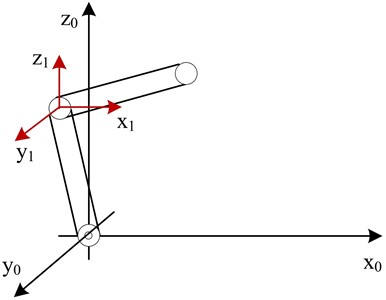After building the simulation model through Simulink, this study first compares the feedforward PD control with the SMC model in this paper. First, a non-interference simulation experiment is carried out. The position tracking curve of robot joint 1 by two control methods is as shown in Fig. 4.

Fig. 4Position curve and error curve of joint 1 of two-joint robot under different control methods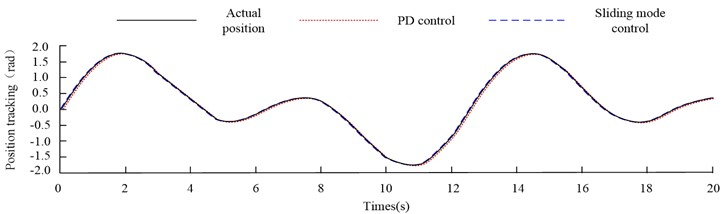a) Position tracking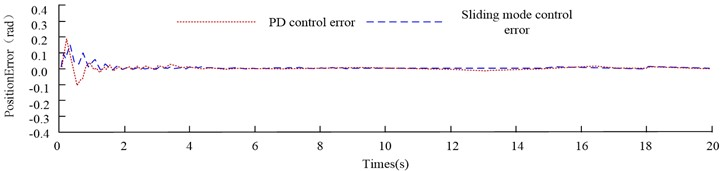b) Position tracking error

From Fig. 4(a), under the two control methods, the two-joint flexible robot achieves stable tracking of the desired position curve after 1.5 s. From Fig. 4(b), when the feedforward PD control mode is adopted, the first joint's maximum inaccuracy is around 0.17 rad.; The maximum error of the first joint under the SMC method is about 0.15 rad. In order to comprehensively evaluate the performance of the control method, the average absolute error (MAE) is used to describe the average error in the experiment. After calculation, the MAE of PD control is 0.02111 rad. The MAE of SMC is 0.0151 rad. From the data, both control methods can reach stable control of the two-joint robot in the non-interference environment. However, the control effect of SMC is better than that of feedforward PD control. In order to analyze the anti-jamming ability of different control methods, this experiment will apply a pulse signal with amplitude of 0.5 rad to the robot's position feedback when the system runs for 10 s, so as to simulate the uncertain interference when the robot runs. Therefore, the control of joint 1 by different control methods in the interference environment is shown in Fig. 5.

Fig. 5Control of joint 1 by different control methods under interference environment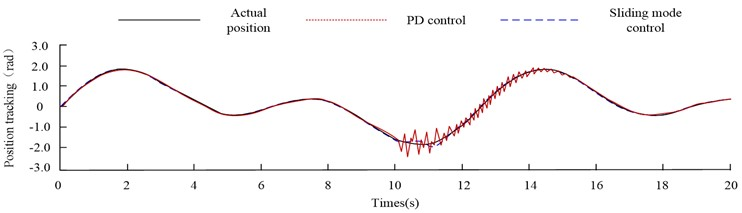a) Position tracking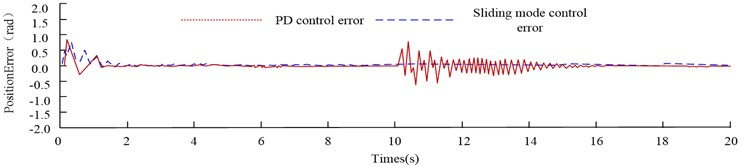b) Position tracking error

From Fig. 5(a), the two control methods have different degrees of error after the pulse interference is applied. The maximum error of the feedforward PD control method reaches 1 rad and the recovery time reaches 5 s. The maximum deviation of the SMC method is only about 0.25 rad; the recovery time is only about 1 s. Therefore, the SMC method has stronger robustness and higher ability to resist uncertain disturbances. Finally, the experimental data of the two joints of the flexible robot are shown in Table 1.

Table 1Comprehensive index value of control method

 Performance index No interference Interference PD control SMC PD control SMC Joint 1 Joint 2 Joint 1 Joint 2 Joint 1 Joint 2 Joint 1 Joint 2 Maximum error (rad) 0.17 0.18 0.15 –0.08 0.75 –1.2 –0.17 0.21 Adjusting time (s) 1.5 1.5 1.5 1.5 5.0 5.0 1.0 1.0 Average absolute error (rad) 0.02111 0.01881 0.0151 0.01413 0.1428 0.2227 0.02313 0.02758

From Table 1, SMC is better than feedforward PD control in terms of maximum system error and average absolute error, and SMC has stronger anti-interference ability. When pulse interference is applied, the recovery time is faster and the error caused is smaller.

### 4.2. Experimental analysis of 6-joint flexible robot under SMC with RBF neural network error compensation

This experiment uses Simscape Multibody to build a 6-DOF cooperative manipulator with flexible joints. In Simscape, the robot dynamics model can be quickly built. In addition, the experiment can simulate the torque sensor, encoder and other components to obtain relevant data. The link mass, gravity acceleration and other information can be defined in the solid model. Simscape can be displayed in the form of simulink module, and then generate animation to observe the operation of the robot. Fig. 6 illustrates the generated robot model.

Fig. 66-DOF robot model with flexible joints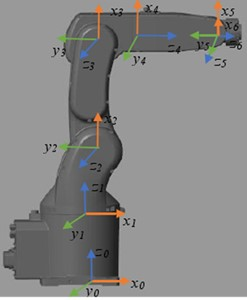As shown in Fig. 6, the 6-joint robot of the experimental object is designed with reference to the human arm joint, which is divided into waist joint (the first joint), shoulder joint (the second joint), elbow joint (the third joint), wrist joint 1 (the fourth joint), wrist joint 2 (the fifth joint) and wrist joint 3 (the sixth joint). Referring to the dynamic model in the study, Table 2 demonstrates the dynamic parameters of the robot.

Table 2Dynamic parameters of flexible 6-joint machine

 Parameter Joint 1 Joint 2 Joint 3 Joint 4 Joint 5 Joint 6 Inertia parameter of connecting rod (mm4) ${I}_{xx}$ 0.000 –1159.074 –411.630 –176.030 64.369 –22.753 ${I}_{yy}$ 0.000 –20399.492 –11.724 –6.067 –3.805 -0.023 ${I}_{zz}$ 3063.912 –18347.709 2135.132 –207.212 57.985 –45.111 ${I}_{xy}$ 0.000 1899.891 –96.343 75.401 –15.400 25.265 ${I}_{xz}$ 0.000 –1430.994 1353.625 –82.863 89.630 -3.642 ${I}_{yz}$ 0.0000 52.088 248.514 –66.656 –103.546 11.230 Centroid position (mm) ${m}_{x}$ 0.000 4516.579 108.279 –77.896 61.692 –4.864 ${m}_{y}$ 0.000 427.753 257.636 –45.278 60.127 24.240 ${m}_{z}$ 0.000 0.000 0.000 0.000 0.000 0.000 Connecting rod mass $m$ 0.001 0.001 0.001 0.001 0.001 0.001 Joint inertia $J$ 0.000 0.000 299.856 447.182 1.812 –181.333 Viscous friction ${f}_{v}$ 13254.743 58605.437 19002.848 4826.342 6055.487 2360.715 Coulomb friction ${f}_{c}$ 10029.767 22852.378 10957.584 3933.646 2879.315 4181.308

In addition to the dynamic parameters of the robot in Table 2, RBF combined with SMC is adopted in this study. The control rate parameters are [3.5×107, 3.5×107, 2.2×107, 3×106, 1.5×106, 1.7×106] T; The sliding surface parameter is [400382300130, 20, 12] T; The sign function coefficient is  T. The radial base width of neurons is 100; The adaptive rate parameter is diag (5, 5, 15, 5, 10, 5). To test the tracking effect of SMC algorithm on different trajectories, 11 path points are selected in Cartesian space to make the end of the manipulator move along the path points. After RBF-sliding mode control planning and PD control planning, the velocity and acceleration of the robot end are shown in Fig. 7.

Fig. 7Velocity and acceleration of robot end under two control methods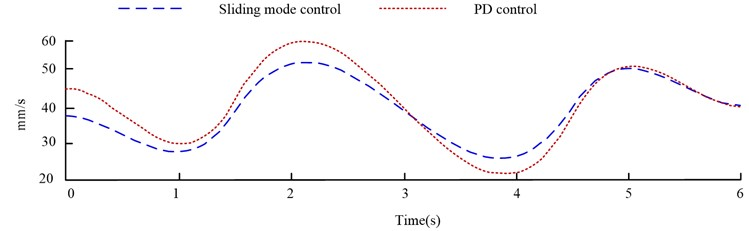a) The speed of robot controlled by different methods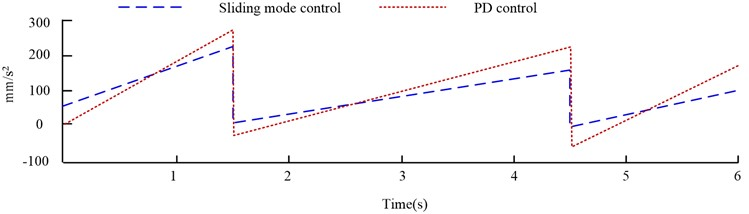b) Acceleration of robot controlled by different methods

From Fig. 7(a), the end velocity of the 6-joint robot is between 30 mm/s and 50 mm/s through RBF-sliding mode control planning; The terminal speed controlled by PD is between 20 mm/s and 60 mm/s. The data shows that the end speed of the robot controlled by RBF - sliding mode is more stable. As can be seen from Fig. 7 (b), when the control rate is switched at a high frequency, both control techniques cause the robot to chatter. PD control robot has discontinuous and non-smooth phenomenon, and also has more obvious jump. The occurrence of this situation will cause a heavy burden on the controller and easily lead to the phenomenon of control instability. At the same time, it will also aggravate the wear of the mechanical arm and other problems. However, the method proposed in the study has a light jump phenomenon and has a better effect on the vibration control of the robot end. From Fig. 8, This research simulates the Cartesian space trajectory of the robot end under the control of the two techniques, as well as observes and analyzes the robot end’s trajectory tracking control effect under the two control methods.

Fig. 8Cartesian space robot trajectory tracking diagram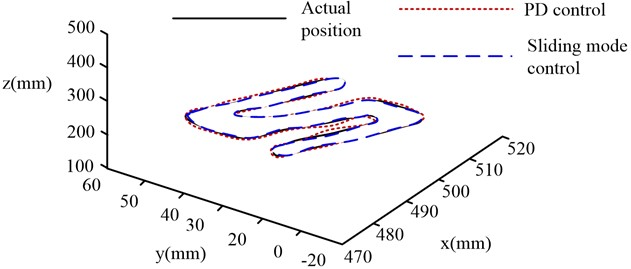From Fig. 8, the two models have better tracking effect on the part of straight line track, and slightly worse tracking effect on the arc track. The reason is that the arc trajectory needs the robot’s centripetal force at each joint to make the robot end complete the arc motion. However, due to the existence of joint flexibility, it is difficult to provide enough centripetal force, which leads to tracking error. From the card in Fig. 8, the maximum tracking error of the traditional PD control exceeds 5 mm, while the maximum error of the optimized Sliding mode control method is within 3 mm. On the whole, the effect of the improved neural network SMC algorithm is better than PD control algorithm. The robustness of SMC overcomes the tracking error caused by joint flexibility to a certain extent. Finally, the three-axis error of the two robot control methods under the trajectory tracking experiment is compared, as shown in Fig. 9.

Fig. 9Three-axis error comparison of different control methods on the end track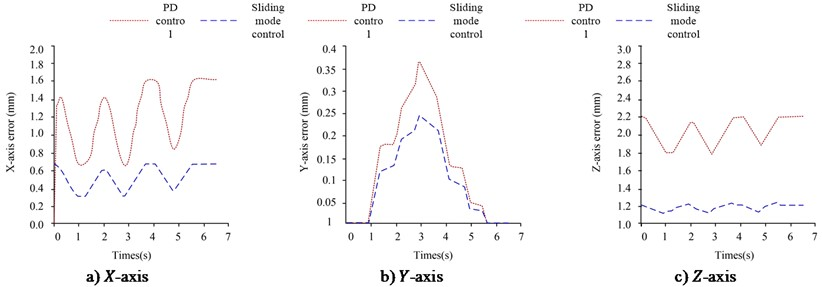From Fig. 9, there are some flaws in the flexible manipulator’s movements in all three directions, and the error of the robot under PD control in the three directions is higher than that of the SMC-RBF method. In Fig. 9(a), the robot’s maximum error end on the $X$-axis under PD control is 1.6 mm; The maximum error of the robot under RBF-sliding mode control is only 0.7 mm; The maximum error of the neural network optimization method on the $X$ axis is 0.9 mm less than that of the traditional method. From Fig. 9(b), the maximum error of the neural network optimization method on the $X$-axis is 0.1mm less than that of the traditional method. The biggest error is 0.25 mm; The biggest error of traditional PD control is 0.35 mm. From Fig. 9(c), the biggest error of the method in this study on the $z$-axis is 1.25 mm; The biggest error of the robot under PD control method is 2.2 mm; After optimization, the maximum error of $z$-axis is reduced by 0.95 mm. Finally, this study uses MAE to comprehensively describe the control error in each direction under the two controls, as shown in Table 3.

Table 3Average absolute error in all directions

 Control method $X$ direction (mm) $Y$ direction (mm) $Z$ direction (mm) PD control 1.219 0.254 1.995 RBF-sliding mode control 0.505 0.092 1.158

From Table 3, the average absolute error of the SMC-RBF in this study on the three axes is 0.505 mm, 0.092 mm and 1.158 mm respectively; The traditional PD control method’s average absolute error on the three axes is 1.219 mm, 0.254 mm and 1.995 mm respectively. It is obviously that the control method that optimizes the SMC method through RBF can effectively weaken the end vibration of the robot.

## 5. Discussion

Flexible robots have many advantages such as low energy consumption, light weight, high load ratio, precise positioning, and less space occupation. They can fundamentally solve the drawbacks of traditional rigid industrial robots and better meet the strict requirements of the aerospace and medical equipment fields, thus making flexible robots play an important role in national defense, industry, and other fields . Although flexible collaborative robots have many advantages, they contain some electronic components that can generate flexibility. These flexible components have low stiffness and are prone to varying degrees of vibration problems during the operation of the robotic arm, which affects the time for the robotic arm to reach steady state, reduces trajectory tracking accuracy, and greatly reduces the performance and work efficiency of the robotic arm . Therefore, improving the control performance of flexible robotic arms and improving control accuracy has always been a widespread concern of many scholars.

At present, PID control, Singular perturbation control and Sliding mode control are the most fruitful. PID control is a common control method for linear system, and it is necessary to improve the parameter tuning method when it is applied to such a nonlinear system as a manipulator [18-19]. The Singular perturbation method is a method of solving nonlinear, high-order differential equations with minimal parameters, which cannot approximate the small parameters to zero. The Singular perturbation method can be used to reduce the order of this higher-order differential equation and decompose it into several small equations to solve them respectively . The sliding mode variable structure method does not need an accurate mathematical model and has strong robustness. It is an effective method to solve the control problems of complex nonlinear systems . Therefore, it is very important to study the Sliding mode control of robot  when considering the uncertain interference and modeling error. This article mainly focuses on the research of vibration suppression at the end of robots with flexible joints, and designs tracking control algorithms for flexible robots from the perspective of vibration suppression. On the basis of Sliding mode control, RBF neural network is used to compensate modeling error online. At the same time, two joint manipulator and six joint manipulator are used as objects for simulation analysis in the experiment. The two degree of freedom flexible manipulator is compared with the manipulator under different control methods to achieve stable tracking of the desired trajectory in 1.5 s. The results show that the tracking error of the optimized Sliding mode control is less than that of other algorithms, At the same time, after the pulse interference is added, the two control methods have different degrees of error. The maximum error of the feedforward PD control method reaches 1 rad, and the recovery time reaches 5 s, while the maximum error of the Sliding mode control method is only about 0.25 rad, and the recovery time only needs about 1s. Therefore, the Sliding mode control method has stronger robustness and higher ability to resist uncertain disturbances.

In the simulation experiment of 6-joint robot, after RBF Sliding mode control planning, the terminal speed of 6-joint robot is between 30 mm/s and 50 mm/s, while the terminal speed of PD control is between 20 mm/s-60 mm/s. In addition, the Cartesian space trajectory of the robot end under the control of the two methods is simulated, and the trajectory tracking control effect of the robot end under the two control methods is observed and analyzed. Finally, the average absolute error of the RBF slicing mode control proposed in this study on the three axes is 0.505 mm, 0.092 mm, and 1.158 mm respectively; The average absolute error of the traditional PD control method on the three axes is 1.219 mm, 0.254 mm and 1.995 mm respectively. The experimental results indicate that the control method for flexible joint robots proposed in this study has higher tracking accuracy and can effectively weaken the robot's chattering.

## 6. Conclusions

To verify the vibration suppression effect of SMC-RBF method in the control of FJB, simulation experiments were carried out on 2-joint flexible robot and 6-joint flexible robot respectively. The simulation experiment on a 2 DOF FJB shows that both methods can make the manipulator reach the state of stable tracking the desired trajectory in 1.5 s. However, the average absolute error of SMC algorithm is about 0.005 rad less than that of feedforward PD control algorithm. When pulse interference with amplitude of 0.5 rad is applied, the recovery time of feedforward PD control algorithm is 5 s; The biggest error is 1 rad. The biggest error of SMC method is only about 0.25 rad; And the recovery time is only about 1s. In the experiment of 6-DOF FJB, the average error in $X$ direction of the robot is 1.219 mm under PD control; The average $Y$-direction inaccuracy is 0.254 mm; The average $Z$ direction inaccuracy is 1.995 mm. Under the effect of improved neural SMC method, only 0.505 mm is the average amount of robot $X$ direction inaccuracy; The biggest error in Y direction is 0.092 mm; The biggest error in $Z$ direction is 1.158 mm. Experiments show that the improved neural SMC has higher tracking accuracy than PD control algorithm. The RBF optimized Sliding mode control method proposed in this study has significant effect on chattering suppression and further improves the control performance of the system. But this method can only weaken chattering and cannot completely eliminate it. The drawback of this experiment is that the study only verified the feasibility of algorithm application and did not analyze the control effect under robot load conditions.

09 March 2023
Accepted
12 June 2023
Published
07 September 2023
SUBJECTS
Vibration control, generation and harvesting
Keywords
vibration control
flexible joint
dynamic model
sliding mode control
RBF neural network
Acknowledgements

The authors have not disclosed any funding.

Data Availability

The datasets generated during and/or analyzed during the current study are available from the corresponding author on reasonable request.

Conflict of interest

The authors declare that they have no conflict of interest.